• 基于小波分析的脑电信号能量特征提取，先对脑电信号进行小波分解，提取小波系数，再用小波系数构造脑电信号的能量特征。
• 于小波变换的脑电信号特征提取 于小波变换的脑电信号特征提取 于小波变换的脑电信号特征提取 于小波变换的脑电信号特征提取
•研究论文
• 针对运动想象脑电信号特征提取困难，分类正确率低的问题，提出了利用小波熵进行特征提取并采用支持向量机（SVM）来分类的算法。计算运动想象脑电信号的功率，通过理论分析选择小波包尺度，对信号功率进行小波包分解...
• 对于癫痫脑电信号的分析都是基于信号本身特征，通过直接的处理，在时域中提取周期和幅值等脑电波形等基本特征作为判断依据。时域分析方法主要是分析脑电信号波形的几何性质，如脑电信号的幅值、方差以及均值等。常用...
1. 时域信号分析
对于癫痫脑电信号的分析都是基于信号本身特征，通过直接的处理，在时域中提取周期和幅值等脑电波形等基本特征作为判断依据。时域分析方法主要是分析脑电信号波形的几何性质，如脑电信号的幅值、方差以及均值等。常用的方法包括相位分析、方差分析、直方图分析、峰值检测、波形参数分析以及线性预测等方法。从直观的癫痫脑电波形参数与正常的脑电信号波形之间分析，可对癫痫发作的可能性进行预测。
2. 频域信号分析（傅里叶变换，功率谱函数）
频域分析方法，主要是通过FFT变换得出信号在频域上的能量变化提取相应脑电特征。经典频域分析法：是以短时间数据的傅氏变换为基础的周期法。将采样的数据作为零处理。但方差特性不好，频率分辨率低。目前功率谱估计是频域分析的主要方法，把幅度随时间变化的时域脑电信号通过变换，转化为随频率变化的脑电功率谱图, 由于癫痫发作时，脑电信号的功率或能量相比未发作时会明显增大，因此将脑电信号的功率谱作为特征能有效区分发作与未发作脑电可定量分析脑电信号各频段的分量,并可直接观察不同脑电节律的分布与变化。
3. 时频域信号分析
时域和频域分析仅仅从一个层面对脑电信号特征进行了反映，且不适合脑电信号这种非平稳的特点，同时对脑电信号的时域和频域特征进行分析更为适合脑电信号研究。时频分析方法清楚地描述了信号频率随时间变化的关系。在非平稳信号分析中，采用的大多都为时频域分析方法，可以将时域和频域两者结合起来，它包括小波变换法，短时傅里叶变换法，匹配追踪法，有限长单位冲激响应滤波等。
4. 非线性分析方法
脑电信号也表现出复杂的非线性动力学特性。通过提取基于非线性动力学理论的脑电特征来识别癫痫脑电已成为癫痫发作自动检测的前沿动向之一。
1）关联维数是时间序列非线性分析中常见的参数,描述了系统在相空间轨迹的维度复杂性,表征了系统的自由度。
2）Lyapunov指数描述了相空间中相邻轨道间收敛或发散的情况,反映了系统对初始值的敏感性。最大Lyapunov指数在脑电记录的不同时期会有显著变化,发作间歇期的最大Lyapunov指数是最高的,但随着发作的临近,最大Lyapunov指数会逐渐下降,在发作期,最大Lyapunov指数达到最低。
5. 多元统计分析方法
脑电信号是一种复杂的信号，它掺杂着许多杂波和干扰信号，如何有效对采集到的脑电信号进行有用信息的提取，滤除不需要的信息，减少处理的冗余性，减少运行时间，多元统计分析方法可以帮我们解决一部分，常使用的方法包括PCA, ICA, LDA等等


展开全文•小波变换
• 首先深入研究了小波变换以及小波方差的计算方法,结合验证脑电图(EEG)存在的ERD/ERS现象,然后利用小波分解系数方差对C3,C4导联脑电信号进行特征提取,最后采用最简线性分类器进行分类,采用分类正确率作为主要评价标准....
• 文章来源于"脑机接口社区"应用深度学习EEGNet来处理脑电信号​mp.weixin.qq.com本篇文章内容主要包括：EEGNet论文；...卷积神经网络(CNN)主要用来自动特征提取和分类，其在计算机视觉和语音识别领域中的使用...
文章来源于"脑机接口社区"应用深度学习EEGNet来处理脑电信号​mp.weixin.qq.com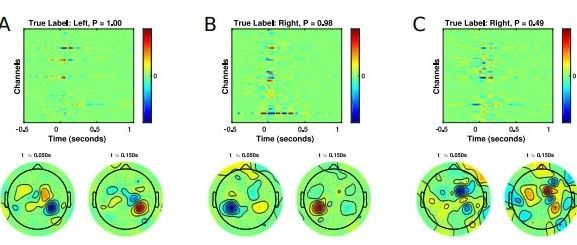本篇文章内容主要包括：EEGNet论文；EEGNet的实现。EEGNet论文简介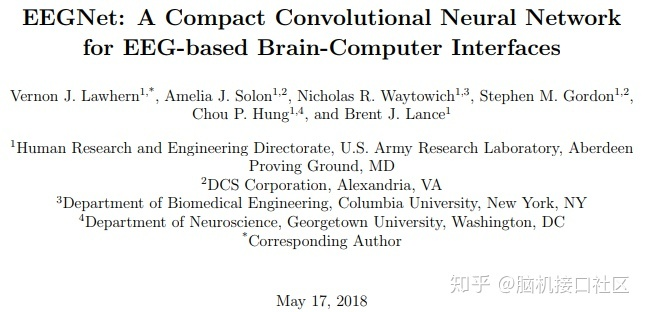脑机接口(BCI)使用神经活动作为控制信号，实现与计算机的直接通信。这种神经信号通常是从各种研究透彻的脑电图(EEG)信号中挑选出来的。卷积神经网络(CNN)主要用来自动特征提取和分类，其在计算机视觉和语音识别领域中的使用已经很广泛。CNN已成功应用于基于EEG的BCI；但是，CNN主要应用于单个BCI范式，在其他范式中的使用比较少，论文作者提出是否可以设计一个CNN架构来准确分类来自不同BCI范式的EEG信号，同时尽可能地紧凑(定义为模型中的参数数量)。该论文介绍了EEGNet，这是一种用于基于EEG的BCI的紧凑型卷积神经网络。论文介绍了使用深度和可分离卷积来构建特定于EEG的模型，该模型封装了脑机接口中常见的EEG特征提取概念。论文通过四种BCI范式(P300视觉诱发电位、错误相关负性反应(ERN)、运动相关皮层电位(MRCP)和感觉运动节律(SMR))，将EEGNet在主体内和跨主体分类方面与目前最先进的方法进行了比较。结果显示，在训练数据有限的情况下，EEGNet比参考算法具有更强的泛化能力和更高的性能。同时论文也证明了EEGNet可以有效地推广到ERP和基于振荡的BCI。网络结构图如下：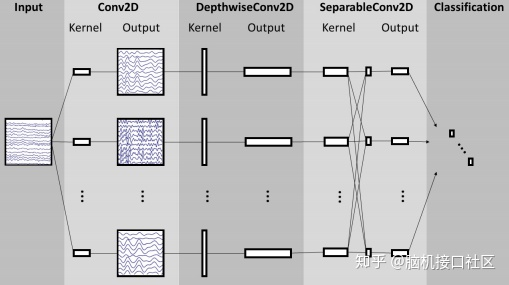实验结果如下图，P300数据集的所有CNN模型之间的差异非常小，但是MRCP数据集却存在显著的差异，两个EEGNet模型的性能都优于所有其他模型。对于ERN数据集来说，两个EEGNet模型的性能都优于其他所有模型(p < 0.05)。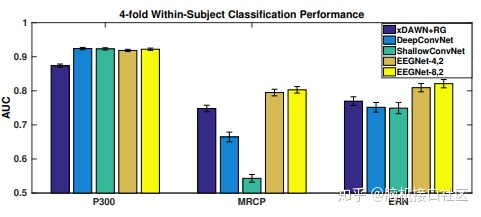如下图每个模型的P300，ERN和MRCP数据集的分类性能平均为30倍。对于P300和MRCP数据集，DeepConvNet和EEGNet模型之间的差异很小，两个模型的性能均优于ShallowConvNet。对于ERN数据集，参考算法（xDAWN + RG）明显优于所有其他模型。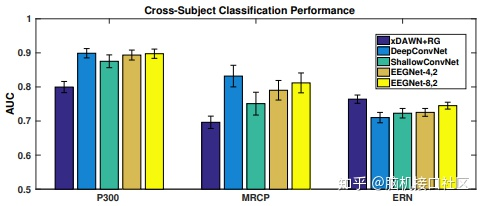下图是对EEGNet-4,1模型配置获得的特征进行可视化。（A）每个空间过滤器的空间拓扑。（B）每个滤波器的目标试验和非目标试验之间的平均小波时频差。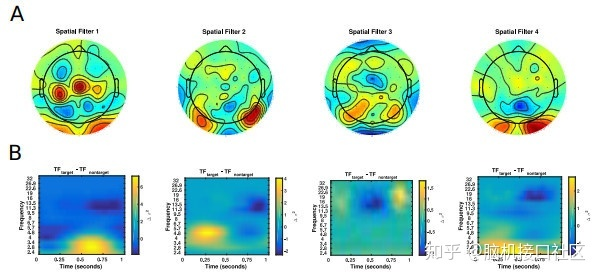下图中第一排是使用DeepLIFT针对MRCP数据集的三个不同测试试验，对使用cross-subject训练的EEGNet-8,2模型进行的单次试验脑电特征相关性：（A）高可信度，正确预测左手运动;（B）高可信度，正确预测右手运动;（C）低可信度，错误预测左手运动。 标题包括真实的类别标签和该标签的预测概率。第二排是在两个时间点的相关性空间分布图：按钮按下后大约50毫秒和150毫秒。与预期的一样，高可信度试验显示出分别对应左（A）和右（B）按钮对应的对侧运动皮层的正确相关性。对于低置信度的试验，可以看到相关性更加混杂且分布广泛，而运动皮质没有明确的空间定位。EEGNet网络实现作者提供的代码用的是旧版本的Pytorch，所以有一些错误。Rose小哥基于作者提供的代码在Pytorch 1.3.1(only cpu)版本下修改，经测试，在Rose小哥环境下可以运行[不排除在其他环境可能会存在不兼容的问题]# 导入工具包
import numpy as np
from sklearn.metrics import roc_auc_score, precision_score, recall_score, accuracy_score
import torch
import torch.nn as nn
import torch.optim as optim
from torch.autograd import Variable
import torch.nn.functional as F
import torch.optim as optimEEGNet网络模型参数如下：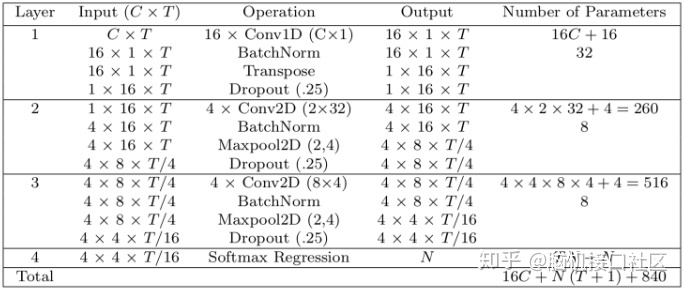定义网络模型：class EEGNet(nn.Module):
def __init__(self):
super(EEGNet, self).__init__()
self.T = 120

# Layer 1
self.conv1 = nn.Conv2d(1, 16, (1, 64), padding = 0)
self.batchnorm1 = nn.BatchNorm2d(16, False)

# Layer 2
self.padding1 = nn.ZeroPad2d((16, 17, 0, 1))
self.conv2 = nn.Conv2d(1, 4, (2, 32))
self.batchnorm2 = nn.BatchNorm2d(4, False)
self.pooling2 = nn.MaxPool2d(2, 4)

# Layer 3
self.padding2 = nn.ZeroPad2d((2, 1, 4, 3))
self.conv3 = nn.Conv2d(4, 4, (8, 4))
self.batchnorm3 = nn.BatchNorm2d(4, False)
self.pooling3 = nn.MaxPool2d((2, 4))

# 全连接层
# 此维度将取决于数据中每个样本的时间戳数。
# I have 120 timepoints.
self.fc1 = nn.Linear(4*2*7, 1)

def forward(self, x):
# Layer 1
x = F.elu(self.conv1(x))
x = self.batchnorm1(x)
x = F.dropout(x, 0.25)
x = x.permute(0, 3, 1, 2)

# Layer 2
x = self.padding1(x)
x = F.elu(self.conv2(x))
x = self.batchnorm2(x)
x = F.dropout(x, 0.25)
x = self.pooling2(x)

# Layer 3
x = self.padding2(x)
x = F.elu(self.conv3(x))
x = self.batchnorm3(x)
x = F.dropout(x, 0.25)
x = self.pooling3(x)

# 全连接层
x = x.view(-1, 4*2*7)
x = F.sigmoid(self.fc1(x))
return x定义评估指标：    acc：准确率    auc:AUC 即 ROC 曲线对应的面积    recall:召回率    precision:精确率    fmeasure：F值def evaluate(model, X, Y, params = ["acc"]):
results = []
batch_size = 100

predicted = []

for i in range(len(X)//batch_size):
s = i*batch_size
e = i*batch_size+batch_size

inputs = Variable(torch.from_numpy(X[s:e]))
pred = model(inputs)

predicted.append(pred.data.cpu().numpy())

inputs = Variable(torch.from_numpy(X))
predicted = model(inputs)
predicted = predicted.data.cpu().numpy()
"""
设置评估指标：
acc：准确率
auc:AUC 即 ROC 曲线对应的面积
recall:召回率
precision:精确率
fmeasure：F值
"""
for param in params:
if param == 'acc':
results.append(accuracy_score(Y, np.round(predicted)))
if param == "auc":
results.append(roc_auc_score(Y, predicted))
if param == "recall":
results.append(recall_score(Y, np.round(predicted)))
if param == "precision":
results.append(precision_score(Y, np.round(predicted)))
if param == "fmeasure":
precision = precision_score(Y, np.round(predicted))
recall = recall_score(Y, np.round(predicted))
results.append(2*precision*recall/ (precision+recall))
return results构建网络EEGNet，并设置二分类交叉熵和Adam优化器# 定义网络
net = EEGNet()
# 定义二分类交叉熵 (Binary Cross Entropy)
criterion = nn.BCELoss()
# 定义Adam优化器
optimizer = optim.Adam(net.parameters())创建数据集"""
生成训练数据集，数据集有100个样本
训练数据X_train:为[0,1)之间的随机数;
标签数据y_train:为0或1
"""
X_train = np.random.rand(100, 1, 120, 64).astype('float32')
y_train = np.round(np.random.rand(100).astype('float32'))
"""
生成验证数据集，数据集有100个样本
验证数据X_val:为[0,1)之间的随机数;
标签数据y_val:为0或1
"""
X_val = np.random.rand(100, 1, 120, 64).astype('float32')
y_val = np.round(np.random.rand(100).astype('float32'))
"""
生成测试数据集，数据集有100个样本
测试数据X_test:为[0,1)之间的随机数;
标签数据y_test:为0或1
"""
X_test = np.random.rand(100, 1, 120, 64).astype('float32')
y_test = np.round(np.random.rand(100).astype('float32'))训练并验证batch_size = 32
# 训练 循环
for epoch in range(10):
print("nEpoch ", epoch)

running_loss = 0.0
for i in range(len(X_train)//batch_size-1):
s = i*batch_size
e = i*batch_size+batch_size

inputs = torch.from_numpy(X_train[s:e])
labels = torch.FloatTensor(np.array([y_train[s:e]]).T*1.0)

# wrap them in Variable
inputs, labels = Variable(inputs), Variable(labels)

# zero the parameter gradients
optimizer.zero_grad()

# forward + backward + optimize
outputs = net(inputs)
loss = criterion(outputs, labels)
loss.backward()

optimizer.step()

running_loss += loss.item()

# 验证
params = ["acc", "auc", "fmeasure"]
print(params)
print("Training Loss ", running_loss)
print("Train - ", evaluate(net, X_train, y_train, params))
print("Validation - ", evaluate(net, X_val, y_val, params))
print("Test - ", evaluate(net, X_test, y_test, params))Epoch 0['acc', 'auc', 'fmeasure']Training Loss 1.6107637286186218Train - [0.52, 0.5280448717948718, 0.6470588235294118]Validation - [0.55, 0.450328407224959, 0.693877551020408]Test - [0.54, 0.578926282051282, 0.6617647058823529]Epoch 1['acc', 'auc', 'fmeasure']Training Loss 1.5536684393882751Train - [0.45, 0.41145833333333337, 0.5454545454545454]Validation - [0.55, 0.4823481116584565, 0.6564885496183207]Test - [0.65, 0.6530448717948717, 0.7107438016528926]Epoch 2['acc', 'auc', 'fmeasure']Training Loss 1.5197088718414307Train - [0.49, 0.5524839743589743, 0.5565217391304348]Validation - [0.53, 0.5870279146141215, 0.5436893203883495]Test - [0.57, 0.5428685897435898, 0.5567010309278351]Epoch 3['acc', 'auc', 'fmeasure']Training Loss 1.4534167051315308Train - [0.53, 0.5228365384615385, 0.4597701149425287]Validation - [0.5, 0.48152709359605916, 0.46808510638297873]Test - [0.61, 0.6502403846153847, 0.5517241379310345]Epoch 4['acc', 'auc', 'fmeasure']Training Loss 1.3821702003479004Train - [0.46, 0.4651442307692308, 0.3076923076923077]Validation - [0.47, 0.5977011494252874, 0.29333333333333333]Test - [0.52, 0.5268429487179488, 0.35135135135135137]Epoch 5['acc', 'auc', 'fmeasure']Training Loss 1.440490186214447Train - [0.56, 0.516025641025641, 0.35294117647058826]Validation - [0.36, 0.3801313628899836, 0.2]Test - [0.53, 0.6113782051282052, 0.27692307692307694]Epoch 6['acc', 'auc', 'fmeasure']Training Loss 1.4722238183021545Train - [0.47, 0.4194711538461539, 0.13114754098360656]Validation - [0.46, 0.5648604269293925, 0.2285714285714286]Test - [0.5, 0.5348557692307693, 0.10714285714285714]Epoch 7['acc', 'auc', 'fmeasure']Training Loss 1.3460421562194824Train - [0.51, 0.44871794871794873, 0.1694915254237288]Validation - [0.44, 0.4490968801313629, 0.2]Test - [0.53, 0.4803685897435898, 0.14545454545454545]Epoch 8['acc', 'auc', 'fmeasure']Training Loss 1.3336675763130188Train - [0.54, 0.4130608974358974, 0.20689655172413793]Validation - [0.39, 0.40394088669950734, 0.14084507042253522]Test - [0.51, 0.5400641025641025, 0.19672131147540983]Epoch 9['acc', 'auc', 'fmeasure']Training Loss 1.438510239124298Train - [0.53, 0.5392628205128205, 0.22950819672131148]Validation - [0.42, 0.4848111658456486, 0.09375]Test - [0.56, 0.5420673076923076, 0.2413793103448276]参考应用深度学习EEGNet来处理脑电信号展开全文• 独立成分分析(ICA)已经成为脑电信号预处理，特别是去除干扰信号过程中一个标准流程。ICA是一种盲源算法，其通过一定的方法把信号分解成相互独立的多个源信号。尽管ICA算法为研究者去除脑电信号中的干扰源提供了便利...


独立成分分析(ICA)已经成为脑电信号预处理，特别是去除干扰信号过程中一个标准流程。ICA是一种盲源算法，其通过一定的方法把信号分解成相互独立的多个源信号。尽管ICA算法为研究者去除脑电信号中的干扰源提供了便利，但是在具体运用时带有一定的主观性，因此需要一定的经验才能够鉴别出干扰成分。当然，目前也有一些自动化鉴别干扰成分插件，但是这些插件也只能提供一个参考而已，最后还需要自己的判定。这里，笔者总结一些典型噪声成分的特点，希望对各位朋友有所帮助。      EEGlab中植入了最常用的ICA算法，建议采用EEGlab运行ICA。ICA跑完之后，可以画出每个成分的拓扑图、功率谱曲线等，我们可以依据这些信息鉴别出噪声成分，进而把这些成分去掉。1.眨眼      眨眼引起的干扰最主要特点是：独立成分的拓扑图主要分布于前端眼部电极，如图1所示。此外，该成分的功率谱曲线没有明显的peak。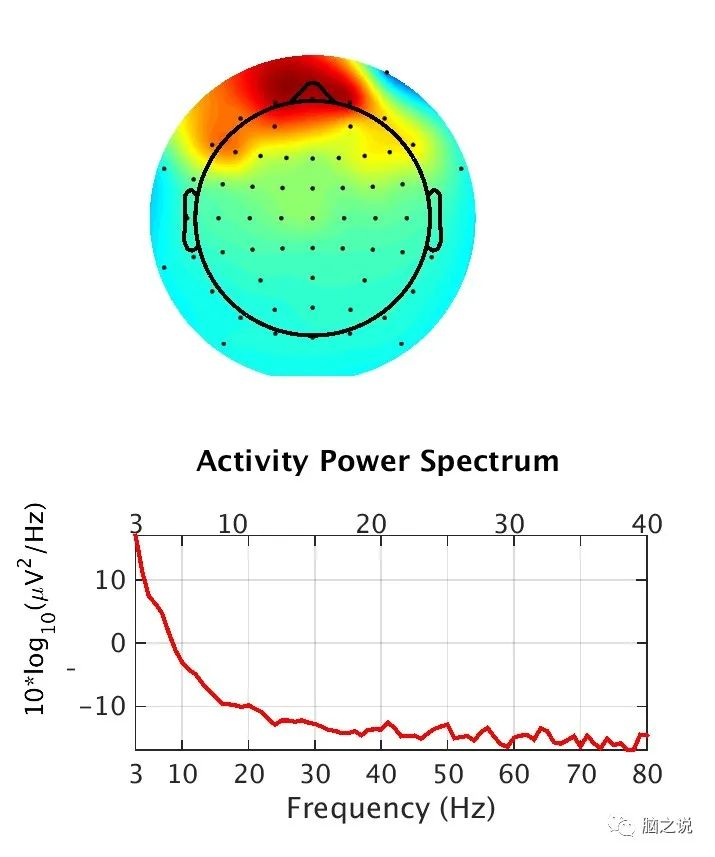图12.肌电       肌电的典型表现是：独立成分拓扑图主要集中在左侧/右侧颞叶处，如图2所示。此外，从功率谱曲线上可以看到，能量主要在高频，这主要是由于肌电一般是高频信号。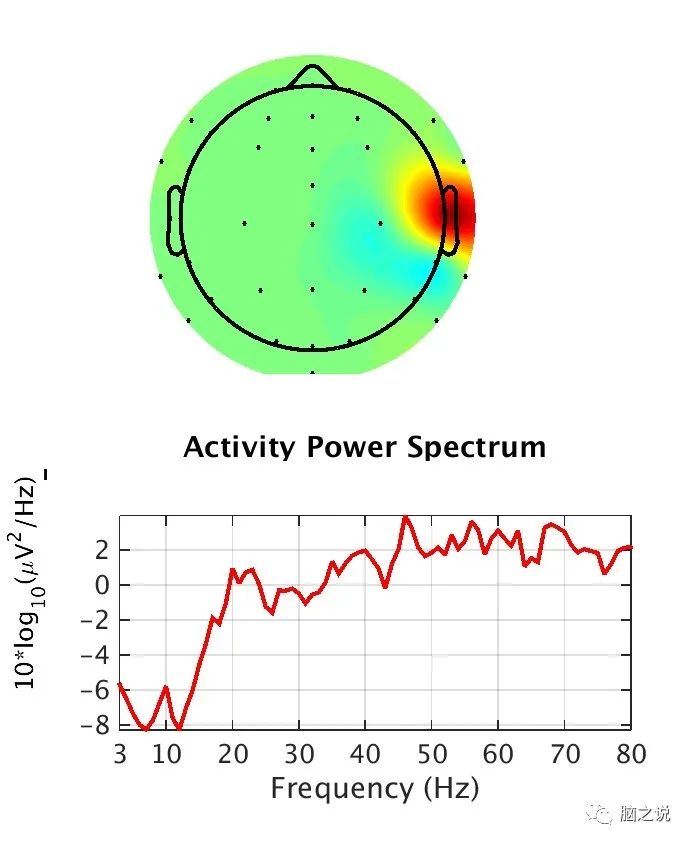图23.通道噪声       通道噪声主要是由于该通道在采集过程中与头皮接触不良引起的，其典型特征是在拓扑图上集中在某一个电极上，如图3所示。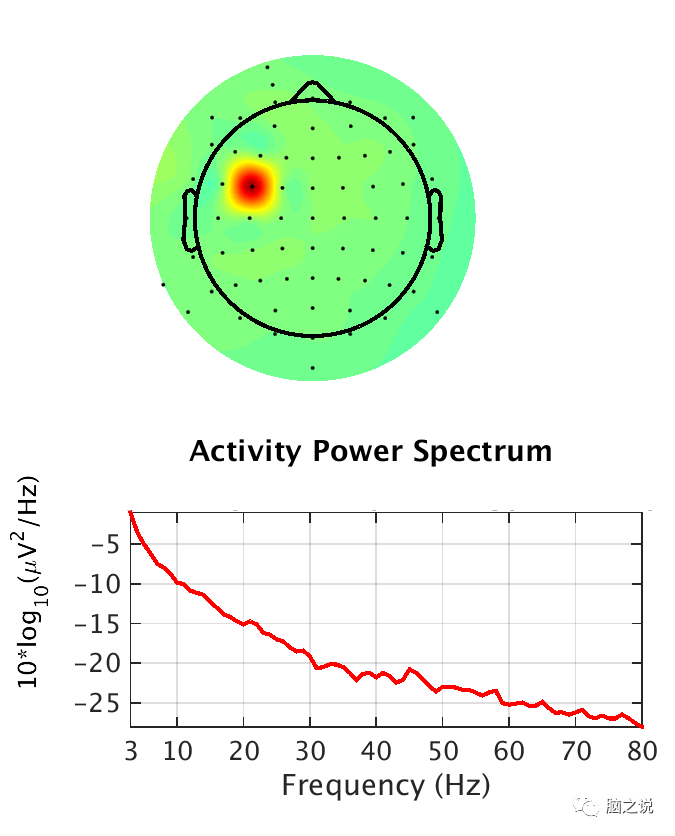图34.心电       心电最明显的特征是该成分的时域信息，可以从该成分的信号中看到明显的心电QRS波，如图4所示。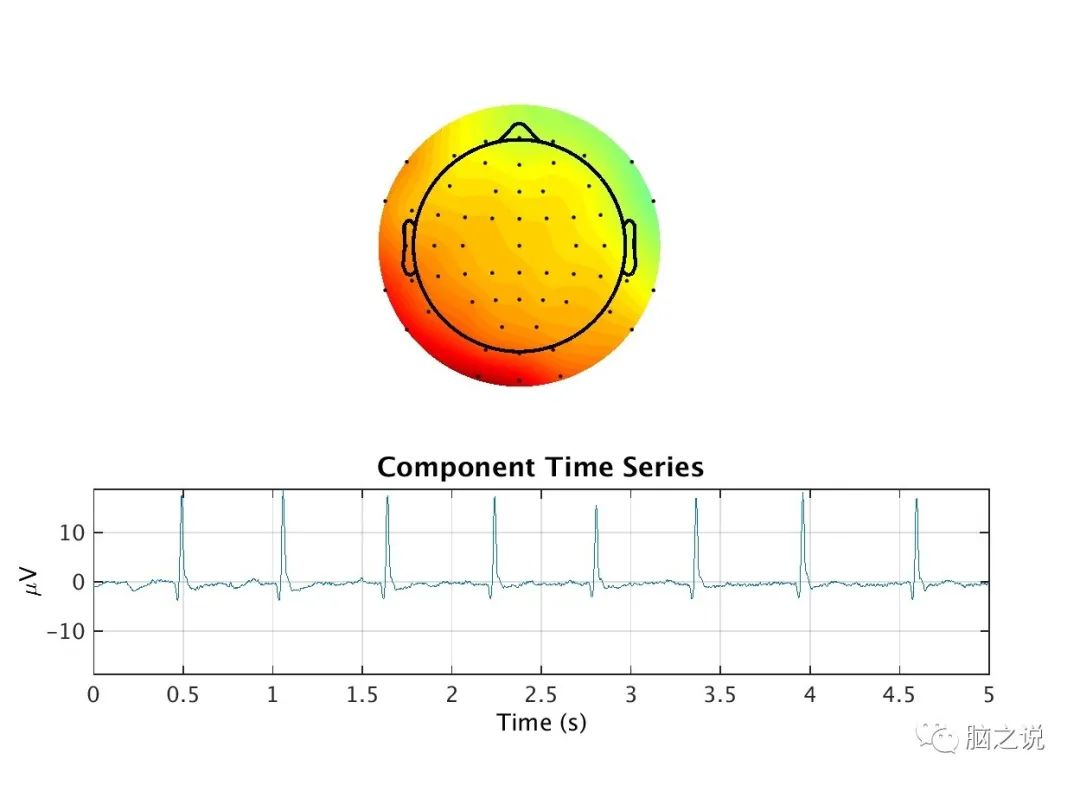图4       当然，上述列举的只是ICA能够鉴别出的最常见的噪声，除此之外，ICA还可以鉴别出工频干扰等噪声。注：原创不易，请多多转发支持，如有问题请加笔者微信交流(悦影科技赵宗亚，微信：15560177218)科研服务第一届脑影像编程技术实战班(郑州，2020.12.19-12.23)第一届脑影像机器学习实战班(郑州，2020.12.24-12.28)脑电数据处理和机器学习分析服务fMRI数据处理服务脑影像数据MVPA和机器学习分析服务结构MRI数据处理服务MRI个性化服务(线上/线下定制课程)关注我们展开全文• 对原始数据处理时无需进行粗粒化提取； 可用于由确定信号与随机信号组成的混合信号。 1、算法步骤 设原始信号为{x(i),i=1,2,…,N}，按照下面公式重构出 m 维向量，用 y（i）表示，{ y(i),i=1,2,…,M,M=N-m+1},即...
二、样本熵
$\Rightarrow$点击此处——Python代码实现

是对近似熵的一种改进算法，是一种不同于近似熵而且不进行自身匹配的统计量方法

近似熵有两个缺点：

近似熵在与自身匹配时具有偏差性；
近似熵结果的一致性较差

样本熵具有如下特点：

具有比时域统计（均值、方差等）更好的估计效果；
对原始数据处理时无需进行粗粒化提取；
可用于由确定信号与随机信号组成的混合信号。

1、算法步骤

设原始信号为{x(i),i=1,2,…,N}，按照下面公式重构出 m 维向量，用 y（i）表示，{ y(i),i=1,2,…,M,M=N-m+1},即：
$y(i)=\{x(i),x(i+1),x(i+2),\cdots ,x(i+m-1)\}$
其中， m 是选定的空间维度矢量，也即窗口长度，表示从第 i 个点开始的连续 m 个值。
计算 y(i)与 y(j)任意分量之间的欧式距离 d{y(i),y(j)}，并将各个分量之间最大的距离定义为最大贡献成分距离 D{y(i),y(j)}，如下式所示：
$D{y(i),y(j)}=max\{|y(i+k)-x(j+k)|\}$
其中，i，j = 1,2, ,N-m+1， k=0,1,…,m-1。
给定阈值 r（r>0），给定嵌入维数 m，计算代表序列{y(i)}规律性的概率大小量度$C_i^m(r)$，即统计 D{y(i),y(j)}<r 的个数$N^m(i)$与总数 N-m+1 的比例，也即概率大小，如公式所示：
KaTeX parse error: Can't use function '$' in math mode at position 9: C_i^m(r)$̲ = \$N^m(i) / (N…
其中， i<=N-m。$N^m(i)$也叫做模板匹配数，其中 j<=N-m。并且对所有的$C_i^m(r)$求平均值：
$B^m(r) = {1 \over {N-m}} (\displaystyle \sum^{N-m}_{i=1} C_i^m(r))$
将嵌入维数设为 m+1，重复步骤（1）到（3），同理得到$B^{m+1}(r)$。所以可以得到该序列的样本熵为:
$SampEn(m,r) = \displaystyle \lim_{N \rightarrow \infty}[-ln{{B^{m+1}(r)} \over {B^m(r)}]}$
若 N 为有限值时，样本熵可为：
$SampEn(m,r) = -ln{{B^{m+1}(r)} \over {B^m(r)}}$

注：一般选取嵌入维数 m=2，有效阈值 r一般选 0.1~0.25 倍的原始数据标准差
2、核心代码

样本熵算法

def maxdist(x_i, x_j):
"""计算矢量之间的距离"""
return np.max([np.abs(np.array(x_i) - np.array(x_j))])

def _std(data):
"""计算标准差"""
if not isinstance(data, np.ndarray):
data = np.array(data)
return np.std(data)

def phi_2(x, m, r):
"""计算熵值"""
length = len(x) - m + 1
# 重构m维向量
y = [x[i:i+m] for i in range(length)]

# 获取所有的比值列表(不与自身匹配。不包括最后一个向量,注意是N-m不是N-m+1)
C = [ len([1 for y_j in y[:-1] if maxdist(y_i, y_j) < r and (y_i != y_j).any()]) / (length-1) for y_i in y[:-1] ]

ans = 0
for i in range(length - 1):
ans += C[i]
return ans / (length - 1)

def Sample_Entropy(data, m):
"""计算样本熵"""
x = data
data_std = _std(x)
r = data_std * 0.15
return - np.log(phi_2(x,m+1,r) / phi_2(x,m,r))


读取数据集

# 使用自定义函数计算样本熵
def solve_2():
calm = []
stress = []
print("calm:")
for i in range(7):
with open('F:\EEG\paper1\calm\s01\_' + channel_names[i] + '.npy', 'rb') as file:
sub = np.load(file, encoding='latin1', allow_pickle=True)
data = sub[0:1000]
ep = Sample_Entropy(data, 2)
calm.append(ep)
print(ep)
print("stress:")
for i in range(7):
with open('F:\EEG\paper1\stress\s01\_' + channel_names[i] + '.npy', 'rb') as file:
sub = np.load(file, encoding='latin1', allow_pickle=True)
data = sub[0:1000]
ep = Sample_Entropy(data, 2)
stress.append(ep)
print(ep)

return calm,stress

calm_3, stress_3 = solve_2()


画图

plt.rcParams['font.sans-serif'] = ['SimHei']  # 用来正常显示中文标签
plt.rcParams['axes.unicode_minus'] = False  # 用来正常显示负号

y = calm_3  # sub
z = stress_3
fig = plt.figure(figsize=(8, 6))

labels = ['FP1', 'F3', 'F7', 'FP2', 'FZ', 'F4', 'F8']

plt.xlabel("导联", fontsize=14)
plt.ylabel("样熵", fontsize=14)

plt.plot(labels, y, c='b', label="平静")
plt.plot(labels, z, c='r', label="压力")
plt.tick_params(axis='both', labelsize=14)
plt.legend()
plt.show()

$\quad$3、参考文献
 苏建新. 基于脑电信号的情绪识别研究[D].
 信号处理算法（2）：样本熵（SampEn）


展开全文机器学习 python
• 设原始信号为{x(i),i=1,2,…,N}，按照下面公式重构出 m 维向量，用 y（i）表示，{ y(i),i=1,2,…,M,M=N-m+1},即： y(i)=x(i),x(i+1),x(i+2),,x(i+m−1)y(i)={x(i),x(i+1),x(i+2), ,x(i+m-1)}y(i)=x(i),x(i+1),x(i+2)...python 机器学习 算法
• 信号时间序列为{X(i),i=1,2,…,n}，进行相空间重构后，得到如下矩阵： [x(1)x(1+τ)⋯x(1+(m−1)τ)x(2)x(2+τ)⋯x(2+(m−1)τ)⋮⋮⋱⋮x(k)x(k+τ)⋯x(k+(m−1)τ)]\left[ \begin{matrix} x(1) & x(1+\tau) &...机器学习 python 算法
• 目录简介EEGNet网络原理EEGNet网络实现 本分享为脑机学习者Rose整理发表于公众号：脑机接口社区(微信号：Brain_Computer).QQ交流群：...卷积神经网络（CNN）主要用来自动特征提取和分类，其在计算机视觉和语音识别领......